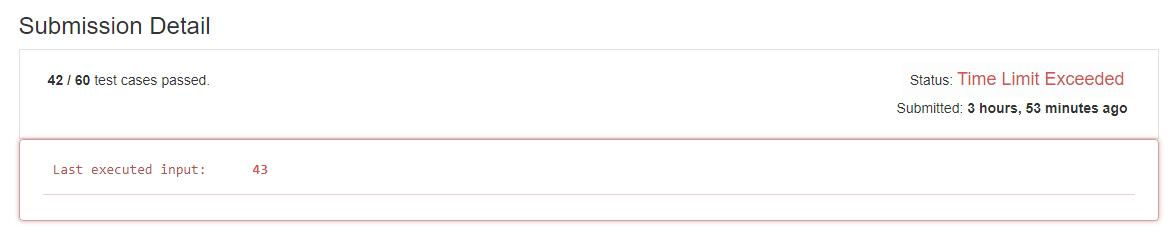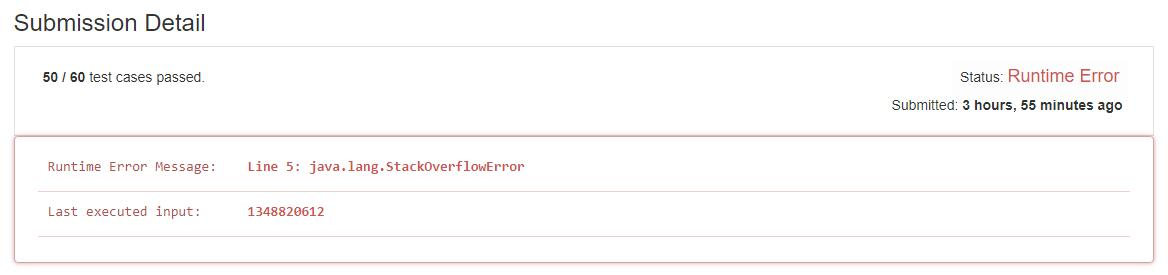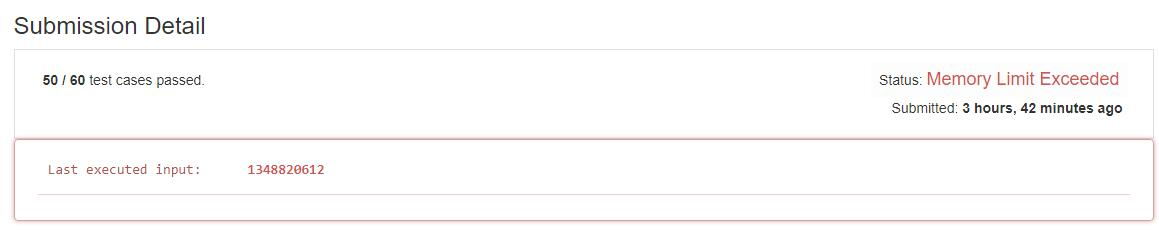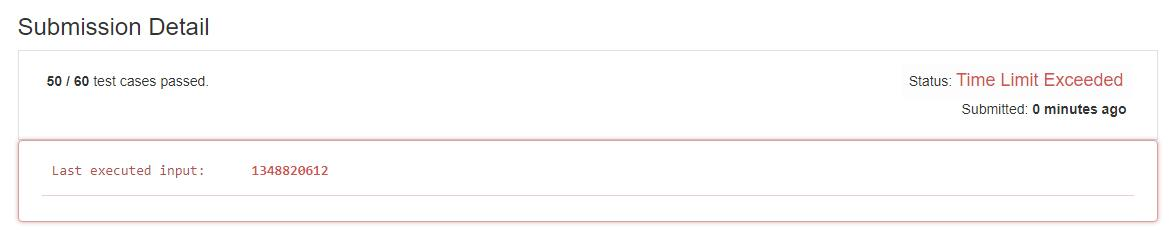# 题目描述（简单难度）

292、Nim Game

You are playing the following Nim Game with your friend: There is a heap of stones on the table, each time one of you take turns to remove 1 to 3 stones. The one who removes the last stone will be the winner. You will take the first turn to remove the stones.

Both of you are very clever and have optimal strategies for the game. Write a function to determine whether you can win the game given the number of stones in the heap.

Example:

Input: 4
Output: false
Explanation: If there are 4 stones in the heap, then you will never win the game;
No matter 1, 2, or 3 stones you remove, the last stone will always be


# 解法一

if (n == 0) {
return false;
}
if (n < 4) {
return true;
}


public boolean canWinNim1(int n) {
if (n == 0) {
return false;
}
if (n < 4) {
return true;
}
//依次尝试拿走 1,2,3 个
for (int i = 1; i <= 3; i++) {
//对方拿走 1 个，2 个，3 个, 你都有稳赢的策略
if (canWinNim(n - i - 1) && canWinNim(n - i - 2) && canWinNim(n - i - 3)) {
return true;
}
}
//否则的话就是你输了
return false;
}HashMap<Integer, Boolean> map = new HashMap<>();
public boolean canWinNim(int n) {
if (map.containsKey(n)) {
return map.get(n);
}
if (n <= 0) {
return false;
}
if (n < 4) {
return true;
}
for (int i = 1; i <= 3; i++) {
if (canWinNim(n - i - 1) && canWinNim(n - i - 2) && canWinNim(n - i - 3)) {
map.put(n, true);
return true;
}
}
map.put(n, false);
return false;
}public boolean canWinNim(int n) {
if(n == 0){
return false;
}
if(n < 4){
return true;
}
boolean[] dp = new boolean[n + 1];
dp = false;
dp = true;
dp = true;
dp = true;
//从下往上走
for (int num = 4; num <= n; num++) {
for (int i = 1; i <= 3; i++) {
if (dp[num - i - 1] && dp[num - i - 2] && dp[num - i - 3]) {
dp[num] = true;
break;
}
}
}
return dp[n];
}public boolean canWinNim(int n) {
if (n == 0) {
return false;
}
if (n < 4) {
return true;
}
boolean[] dp = new boolean;
dp = false;
dp = true;
dp = true;
dp = true;
for (int num = 4; num <= n; num++) {
int i = 1;
for (; i <= 3; i++) {
if (dp[(num - i - 1) % 6] && dp[(num - i - 2) % 6] && dp[(num - i - 3) % 6]){
dp[num % 6] = true;
break;
}
}
if(i == 4){
dp[num % 6] = false;
}
}
return dp[n % 6];
}# 解法二

for (int i = 1; i <= 100; i++) {
System.out.print(canWinNim(i) + " ");
}


true true true false true true true false true true true false true true true false true true true false true true true false true true true false true true true false true true true false true true true false true true true false true true true false true true true false true true true false true true true false true true true false true true true false true true true false true true true false true true true false true true true false true true true false true true true false true true true false true true true false


public boolean canWinNim(int n) {
if (n % 4 == 0) {
return false;
} else {
return true;
}
}


public boolean canWinNim(int n) {
return n % 4 != 0;
}


算法如下：
x&3==0，则是4的倍数。

4　　　　0100
8　　　　1000
12      1100
16     10000
20     10100


public boolean canWinNim(int n) {
return (n & 3) != 0;
}


true true true false true true true false true true true false


n    1    2    3     4    5    6    7     8    ...
true true true false true true true false  ...
n%4  1    2    3     0    1    2    3     0    ...


n        1    2    3    4     5    6    7    8     ...
n-1      0    1    2    3     4    5    6    7     ...
true true true false true true true false  ...
(n-1)%4  0    1    2    3     0    1    2    3     ...


public boolean canWinNim(int n) {
if ((n - 1) % 4 < 3) {
return true;
} else {
return false;
}
}


X X     X X     X X     X X
X X     X X     X X     X X


X X     X X     X X     X X     X
X X     X X     X X     X X


X X     X X     X X     X X     X X
X X     X X     X X     X X


X X     X X     X X     X X     X X
X X     X X     X X     X X     X